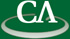## Sparse Polynomial Interpolation.

### Michael Monagan, Department of Mathematics, Simon Fraser University

4:15pm, Thursday August 12th, 2010, in IRMACS.

```Abstract:

Let f be a polynomial in R[x1,x2,...,xn] of degree d with t non-zero terms
which is represented as a "black box", i.e., all we can do is evaluate f
at a point (a1,a2,...,an) in R^n.  We call this ``probing'' the black
box and we seek algorithms to interpolate f which minimize the number of
probes (values).  Consider

f = 1 + x1^d + x2^d + ... + xn^d

We can interpolate f from (d+1)^n values (probes) using Newton interpolation.
But this is exponential in n and since multivariate polynomials in practice
are usually sparse, that is, t << (d+1)^n, we seek algorithms which
do a polynomial number of probes in t, n and d.

In this talk we will present several previous methods, including
o Richard Zippel's probabilistic method for R = Zp from 1979,
o Ben-Or and Tiwari's deterministic method from 1988 for R = Z,
o Huang and Rao's parallel method for small finite fields from 1999,
o Kaltofen, Lee and Lobo's racing algorithm from 2000 for R = Zp.
Zippel's algorithm is used by Maple, Magma and Mathemtica for
polynomial GCD computation over Z.  It makes O(ndt) probes.
For algorithms which need to interpolate over R = Zp, Kaltofen,
Lee and Lobo's method is the most efficient method (makes O(nt) probes)
but it is highly sequential.  We present a new parallel method
which attains the same (O(nt) values).  We have present also
benchmarks comparing our algorithm with Zippel's.

This is joint work with Mahdi Javadi.
```